I.3.4

4-8-2021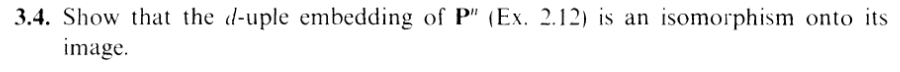Okay, let's get to the exercise. Remember 2.12? ...Looking back, I'm seeing some fun typos, including the symbol ρ magically switching to ϕ midway through (... srsly, I really like writing and typing ϕ so I gravitate towards it). On the other hand, thank fucking goodness I maintain this fucking blog. Coincidentally, I actually talked about this at the end of that exercise (*blows kiss*), but honestly doing this exercise would have been horrific had I had to rely on leafing through my messy notes.

I'm going to be assuming familiarity with 2.12 moving forward. Back in that exercise, we had defined the d-uple embedding

 ρ : Pn → PN (…,xi,…)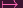(…,Mi(x),…)

We showed that ρ induced a homeomorphism Pn Z(α), which in fact corresponded to the map of coordinate rings

 θ : k[y0,…,yN] → k[x0,…,xn] yi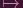Mi

(correspondingly inducing k[y0,,yN]∕α k[x0,,xn])

Remember: Unless our varieties are affine, we can't assume that isomorphic coordinate rings give us isomorphic varieties. Otherwise this exercise would be trivial. We have to show manually that ρ and its inverse satisfies the regularity properties.

Let's start with ρ. Give me some V open in Z(α), and f : V k regular. I need to show that f ρ : ρ-1(V ) k is regular.

Given Y V , we know that there is some U open in V containing Y such that f = g∕h on U (g,h k[y0,,yN], h nowhere zero on U). btw, everytime I have to do this regular thing, I have to write out this crazy long setup. So annoying. You might see me shorten or omit some of it in the future..... perhaps in this very exercise!

Given X ρ-1(U), note that

 f ∘ ρ(X) = f(ρ(X)) =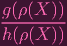=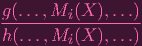=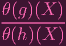And note that for θ(h)(X) = 0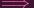h(ρ(X)) = 0, which is a contradiction since X ρ-1(U)ρ(X) Uh(ρ(X))0, so θ(h) is nowhere zero on ρ-1(U), and f ρ = θ(g)∕θ(h) on ρ-1(U).

Since Y was arbitrary, the corresponding ρ-1(U)s clearly cover ρ-1(V ), so f is therefore regular.

Hence, ρ is confirmed a morphism. Now we have to show it for ρ-1

How is ρ-1 defined? Well, with the appropriate permutation of the Mis, my 2.12 work tells me that it's given by (X0,,Xn) where

 X0 = (Y 0)1g∕d (where, remember I can choose ANY of the roots) X1 = Y 1g∕(X0d-1) X2 = Y 2g∕(X0d-1)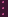Xi = Y ig∕(X0d-1)Xn = Y ng∕(X0d-1)

(again, thank fucking goodness I maintain this blog. With my organization skills, this would have been hidden in one of my three notebooks in opaque context)

By the way, isn't it weird that all those coordinates have analogous definitions except X0. Don't you think, X0 could be put in the same format?

You see, if I write everything in terms of Y 0 (which I'll need to do soon, anyway), I can write:

 X0 = (Y 0)1∕d X1 = Y 1∕(Y 0(d-1)∕d) X2 = Y 2∕(Y 0(d-1)∕d)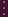Xi = Y i∕(Y 0(d-1)∕d)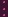Xn = Y n∕(Y 0(d-1)∕d)

Of course, this suggests another definition for X0. Let's confirm (...sorry, those fractions are supposed to be in the exponent. It came out weird):

 Y 0∕(Y 0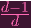) = Y 0 ⋅ (Y 0-) = Y 01-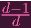= Y 0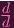-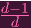= Y 0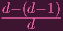= Y 0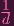Hence we can write X0 = Y 0(Y 0(d-1)∕d). So, in general, the map ρ-1 is given by

 Xi = Y i∕(Y 0(d-1)∕d)

(i = 0,,n)

Oh.... Since they're all multiplied by the same constant, and we're in projective space, I can actually just write

 Xi = Y i

So... ρ-1 is actually just "keep the coordinates 0 through n and drop the rest" I.e., it's given by

 YiY i (i = 0,…,n)

... That's a huge improvement over my initial definition...

Okay, with this nice definition of ρ-1, let's confirm the regularity property. Given V open in Pn, and f : V k regular, we want to verify that f ρ-1 : ρ(V ) k is regular.

Suppose f = g∕h on an open U of V , h nowhere zero on U. Consider some Y ρ(U). Then...

 f ∘ ρ-1(Y ) =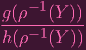=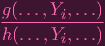(now you perhaps see why I did all that work to rewrite ρ-1). Again, h(,Y i,) = 0h(ρ-1(X)) = 0 yielding a contradiction, so h is nowhere zero on ρ(U). And although g,h came from k[x0,,xn], we can interpret them as elements of k[y0,,yN], so g∕h gives f ρ-1 as a rational function on ρ-1(U). Since U was arbitrary, f ρ-1 is regular on ρ-1(V ) DUN.

It's been a while since I've uploaded in consecutive days.... Perhaps I can keep it up? What's that? Those strange letters are still written on my lips? Well, reader, you know what to do ... muahahahahaha, with a strong "muah".